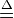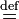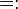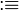# User:IssaRice/Colon-equals allows directionality

For definitions, I prefer$:=$ over other notations like$\equiv$,$\overset{\Delta}{=}$, and$\overset{\text{def}}{=}$. One reason is that$:=$ has the counterpart$=:$ that allows one to place the definiendum on the right. This allows one to e.g. write some long expression (during a calculation), then quickly assign it to something temporarily.
Leary & Kristiansen's logic textbook uses$:\equiv$ in the meta-level language.